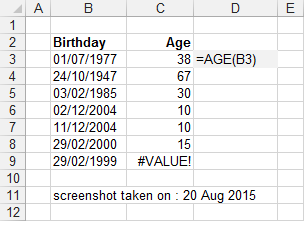# AGE

Returns the age of a person given a birthday.

### Remarks

* For instructions on how to add a function to a workbook refer to the page under Inserting Functions
* The equivalent JavaScript function is AGEIf "dtBirthday" is not a valid date , then #VALUE! is returned.
Alternatively you could use the following formula: =INT(YEARFRAC(TODAY(),"01/07/1977",1))

`'dtBirthday - The date of the birthday.Public Function AGE(ByVal dtBirthday As Date) As Integer    If Int(dtBirthday) > 0 Then       Select Case Month(Date())          Case Is < Month(dtBirthday)                AGE = Year(Date()) - Year(dtBirthday) - 1          Case Is = Month(dtBirthday)             If Day(Date) >= Day(dtBirthday) Then                AGE = Year(Date()) - Year(dtBirthday)             Else                AGE = Year(Date()) - Year(dtBirthday) - 1             End If          Case Is > Month(dtBirthday)             AGE = Year(Date()) - Year(dtBirthday)       End Select    Else       AGE = CVErr(xlErrNA)    End If End Function `